# (F) Concept "I can ... statements"

 Interpreting Functions Building Functions Linear, Quadratic, and Exponential Models Trigonometric Functions MAT-HS: Function | MAT-HS: Statistics and ProbabilitySearch full text

ALL

Page:  1  2  3  4  (Next)
ALL

### M

#### MAT-HS.F-BF.01

 MAT-HS Targeted Standards(F) Concept: Function(BF) Domain: Building FunctionsCluster: Build a function that models a relationship between two quantities MAT-HS.F-BF.01 Write a function that describes a relationship between two quantities. a. Determine an explicit expression, a recursive process, or steps for calculation from a context. b. Combine standard function types using arithmetic operations.For example, build a function that models the temperature of a cooling body by adding a constant function to a decaying exponential, and relate these functions to the model. c. Compose functions. For example, if T(y) is the temperature in the atmosphere as a function of height, and h(t) is the height of a weather balloon as a function of time, then T(h(t)) is the temperature at the location of the weather balloon as a function of time.

## Student Learning Targets:

### Knowledge Targets

• I can recognize the notation for the composition of functions.
• I can recognize the proper notation for inverse functions.

### Reasoning Targets

• I can determine the inverse of a function.
• I can interpret the results of the horizontal line test.
• I can verify whether two functions are inverses of each other using compositions.

### Skills (Performance) Targets

• I can graph a linear equation.
• I can manipulate the form of a linear equation.
• I can identify a linear equation.
• I can use algebraic expressions, equations or inequalities involving one or two variables to represent geometric relationships.
• I can perform composition of algebraic functions.
• I can demonstrate the inverse of an algebraic function graphically.
• I can perform the horizontal line test to determine if the inverse of a function exists.
• I can combine function types, such as linear and exponential, using arithmetic operations.

### Product Targets

• I can write a functions that describes a relationship between two quantities.
• I can write an explicit or recursive expression or describe the calculations needed to model a function given a situation.

## Proficiency Scale

 Score Description Sample Activity 4.0 In addition to Score 3.0, the student demonstrates in-depth inferences and applications regarding more complex material that go beyond end of instruction expectations. - 3.5 In addition to Score 3.0 performance, the student demonstrates in-depth inferences and applications regarding the more complex content with partial success. 3.0 “The Standard.” The student demonstrates no major errors or omissions regarding any of the information and processes that were end of instruction expectations. - 2.5 The student demonstrates no major errors or omissions regarding the simpler details and processes (Score 2.0 content) and partial knowledge of the more complex ideas and processes (Score 3.0 content). 2.0 The student demonstrates no major errors or omissions regarding the simpler details and processes but exhibits major errors or omissions regarding the more complex ideas and processes (Score 3.0 content). - 1.5 The student demonstrates partial knowledge of the simpler details and processes (Score 2.0 content) but exhibits major errors or omissions regarding the more complex ideas and procedures (Score 3.0 content). 1.0 With help, the student demonstrates a partial understanding of some of the simpler details and processes (Score 2.0 content) and some of the more complex ideas and processes (Score 3.0 content). - 0.5 With help, the student demonstrates a partial understanding of some of the simpler details and processes (Score 2.0 content) but not the more complex ideas and processes (Score 3.0 content). 0.0 Even with help, the student demonstrates no understanding or skill. -

## Resources

 Web Vocab explicit recursive linear exponential function

#### MAT-HS.F-BF.02

 MAT-HS Targeted Standards(F) Concept: Function(BF) Domain: Building FunctionsCluster: Build a function that models a relationship between two quantities MAT-HS.F-BF.02 Write a function that describes a relationship between two quantities.

## Student Learning Targets:

• I can
• I can

• I can
• I can

### Skills (Performance) Targets

• I can make connections between linear functions and arithmetic sequences, and exponential functions and geometric sequences.

### Product Targets

• I can write and translate between the recursive and explicit formula for a arithmetic sequence and use the formulas to model a situation.
• I can write and translate between the recursive and explicit formula for a geometric sequence and use the formulas to model a situation.

## Proficiency Scale

 Score Description Sample Activity 4.0 In addition to Score 3.0, the student demonstrates in-depth inferences and applications regarding more complex material that go beyond end of instruction expectations. - 3.5 In addition to Score 3.0 performance, the student demonstrates in-depth inferences and applications regarding the more complex content with partial success. 3.0 “The Standard.” The student demonstrates no major errors or omissions regarding any of the information and processes that were end of instruction expectations. - 2.5 The student demonstrates no major errors or omissions regarding the simpler details and processes (Score 2.0 content) and partial knowledge of the more complex ideas and processes (Score 3.0 content). 2.0 The student demonstrates no major errors or omissions regarding the simpler details and processes but exhibits major errors or omissions regarding the more complex ideas and processes (Score 3.0 content). - 1.5 The student demonstrates partial knowledge of the simpler details and processes (Score 2.0 content) but exhibits major errors or omissions regarding the more complex ideas and procedures (Score 3.0 content). 1.0 With help, the student demonstrates a partial understanding of some of the simpler details and processes (Score 2.0 content) and some of the more complex ideas and processes (Score 3.0 content). - 0.5 With help, the student demonstrates a partial understanding of some of the simpler details and processes (Score 2.0 content) but not the more complex ideas and processes (Score 3.0 content). 0.0 Even with help, the student demonstrates no understanding or skill. -

## Resources

 Web Vocab sequence arithmetic sequences geometric sequences

#### MAT-HS.F-BF.03

 MAT-HS Targeted Standards(F) Concept: Function(BF) Domain: Building FunctionsCluster: Build new functions from existing functions. MAT-HS.F-BF.03*   Identify the effect on the graph of replacing f(x) by f(x) + k, f(x + k), k f(x), and f(kx), for specific values of k (both positive and negative); find the value of k given the graphs. Recognize even and odd functions from their graphs.

## Student Learning Targets:

• I can
• I can

• I can
• I can

### Skills (Performance) Targets

• I can experiment to identify, using technology, the transformational effects on the graph of a function f(x) when f(x) is replaced by f(x)+k, k∙f(x), f(kx), and f(x+k) for specific values of k, both positive and negative.
• I can find the value of k given the graph of a transformed function.
• I can recognize even and odd functions from their graphs and equations.

• I can
• I can

## Proficiency Scale

 Score Description Sample Activity 4.0 (advanced) In addition to Score 3.0, the student demonstrates in-depth inferences and applications regarding more complex material that go beyond end of instruction expectations. Write three different quadratic functions whose graphs have the line x = 4 as an axis of symmetry but have different y-intercepts. 3.5 In addition to Score 3.0 performance, the student demonstrates in-depth inferences and applications regarding the more complex content with partial success. 3.0 (proficient) The student can: analyze the transformational effects on the graph of a function. find the value of k given the graph of a transformed function. determine whether a function is even or odd given a graph. The student exhibits no major errors or omissions. Describe all the transformations and graph the function.2.5 The student demonstrates no major errors or omissions regarding the simpler details and processes (Score 2.0 content) and partial knowledge of the more complex ideas and processes (Score 3.0 content). 2.0 (progressing) There are no major errors or omissions regarding the simpler details and processes as the student can: recognize and recall specific terminology, such as: vertical shift horizontal shift compress/amplified stretch/shrink reflection even function odd function  However, the student exhibits major errors or omissions regarding the more complex ideas and processes. Given the graph, identify all of the transformations.1.5 The student demonstrates partial knowledge of the simpler details and processes (Score 2.0 content) but exhibits major errors or omissions regarding the more complex ideas and procedures (Score 3.0 content). 1.0 (beginning) With help, the student demonstrates a partial understanding of some of the simpler details and processes (Score 2.0 content) and some of the more complex ideas and processes (Score 3.0 content). 0.5 With help, the student demonstrates a partial understanding of some of the simpler details and processes (Score 2.0 content) but not the more complex ideas and processes (Score 3.0 content). 0.0 Even with help, the student demonstrates no understanding or skill.

## Resources

 Web Vocab transformation even functions odd functions

#### MAT-HS.F-BF.04

 MAT-HS Targeted Standards(F) Concept: Function(BF) Domain: Building FunctionsCluster: Build new functions from existing functions MAT-HS.F-BF.04 Find inverse functions. a. Solve an equation of the form f(x) = c for a simple function f that has an inverse and write an expression for the inverse.For example, f(x) =2 x³ or f(x) = (x+1)/(x-1) for x ≠ 1. b. Verify by composition that one function is the inverse of another. c. Read values of an inverse function from a graph or a table, given that the function has an inverse. d. Produce an invertible function from a non-invertible function by restricting the domain.

## Student Learning Targets:

### Knowledge Targets

• I can recognize the notation for the composition of functions.
• I can recognize the proper notation for inverse functions.

### Reasoning Targets

• I can determine the inverse of a function.
• I can interpret the results of the horizontal line test.
• I can verify whether two functions are inverses of each other using compositions.

### Skills (Performance) Targets

• I can perform composition of algebraic functions.
• I can demonstrate the inverse of an algebraic function graphically.
• I can perform the horizontal line test to determine if the inverse of a function exists.
• I can find the inverse of a given function.
• I can solve a function for the dependent variable and write the inverse of a function by interchanging the dependent and independent variables.

• I can
• I can

## Proficiency Scale

 Score Description Sample Activity 4.0 In addition to Score 3.0, the student demonstrates in-depth inferences and applications regarding more complex material that go beyond end of instruction expectations. - 3.5 In addition to Score 3.0 performance, the student demonstrates in-depth inferences and applications regarding the more complex content with partial success. 3.0 “The Standard.” The student demonstrates no major errors or omissions regarding any of the information and processes that were end of instruction expectations. - 2.5 The student demonstrates no major errors or omissions regarding the simpler details and processes (Score 2.0 content) and partial knowledge of the more complex ideas and processes (Score 3.0 content). 2.0 The student demonstrates no major errors or omissions regarding the simpler details and processes but exhibits major errors or omissions regarding the more complex ideas and processes (Score 3.0 content). - 1.5 The student demonstrates partial knowledge of the simpler details and processes (Score 2.0 content) but exhibits major errors or omissions regarding the more complex ideas and procedures (Score 3.0 content). 1.0 With help, the student demonstrates a partial understanding of some of the simpler details and processes (Score 2.0 content) and some of the more complex ideas and processes (Score 3.0 content). - 0.5 With help, the student demonstrates a partial understanding of some of the simpler details and processes (Score 2.0 content) but not the more complex ideas and processes (Score 3.0 content). 0.0 Even with help, the student demonstrates no understanding or skill. -

## Resources

 Web Inverse Functions WS: Vocab inverse function independent variable dependent variable

#### MAT-HS.F-BF.05

 MAT-HS Targeted Standards(F) Concept: Function(BF) Domain: Building FunctionsCluster: Build new functions from existing functions MAT-HS.F-BF.05 Understand the inverse relationship between exponents and logarithms and use this relationship to solve problems involving logarithms and exponents.

• I can
• I can

• I can
• I can

• I can
• I can

• I can
• I can

## Alg II Solve Logarithmic Equations Proficiency Scale

 Score Description Sample Activity 4.0 (advanced) In addition to Score 3.0, the student demonstrates in-depth inferences and applications regarding more complex material that go beyond end of instruction expectations. Describe and correct the error in this work: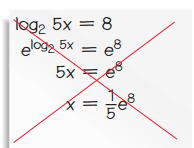3.5 In addition to Score 3.0 performance, the student demonstrates in-depth inferences and applications regarding the more complex content with partial success. 3.0 (proficient) The student can: solve a logarithmic equation and recognize an extraneous solution. The student exhibits no major errors or omissions. Solve the following logarithmic equation and check for extraneous solutions: log3(x2-30)=log3(x)    Solve the following logarithmic equation and check for extraneous solutions: 2log2(x)=log2(4)+log2(x-1) 2.5 The student demonstrates no major errors or omissions regarding the simpler details and processes (Score 2.0 content) and partial knowledge of the more complex ideas and processes (Score 3.0 content). 2.0 (progressing) There are no major errors or omissions regarding the simpler details and processes as the student can: recognize and recall specific terminology such as: Base Logarithm Exponentiate Condense Expand solve simple logarithmic equations. evaluate a logarithmic expression. use properties of logarithms to expand and condense an expression.  However, the student exhibits major errors or omissions regarding the more complex ideas and processes. Solve the following logarithmic equation for x: log10(100)=x 1.5 The student demonstrates partial knowledge of the simpler details and processes (Score 2.0 content) but exhibits major errors or omissions regarding the more complex ideas and procedures (Score 3.0 content). 1.0 (beginning) With help, the student demonstrates a partial understanding of some of the simpler details and processes (Score 2.0 content) and some of the more complex ideas and processes (Score 3.0 content). - 0.5 With help, the student demonstrates a partial understanding of some of the simpler details and processes (Score 2.0 content) but not the more complex ideas and processes (Score 3.0 content). 0.0 Even with help, the student demonstrates no understanding or skill. -

## Resources

 Web Vocab

#### MAT-HS.F-IF.01

 MAT-HS Targeted Standards(F) Concept: Function(IF) Domain: Interpreting FunctionsCluster: Understand the concept of a function and use function notation. MAT-HS.F-IF.01 Understand that a function from one set (called the domain) to another set (called the range) assigns to each element of the domain exactly one element of the range. If f is a function and x is an element of its domain, then f(x) denotes the output of f corresponding to the input x. The graph of f is the graph of the equation y = f(x).

## Student Learning Targets:

### Knowledge Targets

• I can identify that the graph of the function f is the graph of the function y=f(x).
• I can identify x as an element of the domain and f(x) as an element in the range given the function f.

• I can
• I can

### Skills (Performance) Targets

• I can use the definition of a function to determine whether a relationship is a function given a table, graph or words.

• I can
• I can

## Proficiency Scale

 Score Description Sample Activity 4.0 In addition to Score 3.0, the student demonstrates in-depth inferences and applications regarding more complex material that go beyond end of instruction expectations. - Graph the following and determine if it is a function.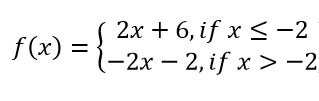3.5 In addition to Score 3.0 performance, the student demonstrates in-depth inferences and applications regarding the more complex content with partial success. 3.0 The student can: understand that a function from one set (called the domain) to another set (called the range) assigns to each element of the domain exactly one element of the range. If f is a function and x is an element of its domain, then f(x) denotes the output of f corresponding to the input x. The graph of f is the graph of the equation y = f(x). The student exhibits no major errors or omissions. Is the relation a function (yes or no)? Explain. a.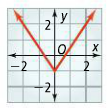b.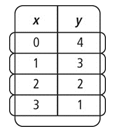Identify the domain and range of the relation. Is this relation a function (yes or no)?  Explain. (-2, 0.5), ( 0,2.5), (4,6.5), (5,2.5) Graph f (x) = 2x + 2 2.5 The student demonstrates no major errors or omissions regarding the simpler details and processes (Score 2.0 content) and partial knowledge of the more complex ideas and processes (Score 3.0 content). 2.0 There are no major errors or omissions regarding the simpler details and processes as the student: Recognize and recall basic vocabulary such as:range domain relation function notation vertical line test However, the student exhibits major errors or omissions regarding the more complex ideas and processes. Find the range of the relation.(4, -2), (-2, 3), (1, -3). Find the domain of the relation. (2, 4), (8, 11), (9, 1), (4, 2) Does the relation pass a vertical line test?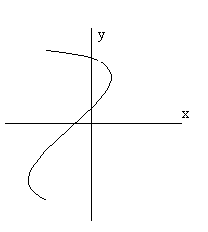1.5 The student demonstrates partial knowledge of the simpler details and processes (Score 2.0 content) but exhibits major errors or omissions regarding the more complex ideas and procedures (Score 3.0 content). 1.0 With help, the student demonstrates a partial understanding of some of the simpler details and processes (Score 2.0 content) and some of the more complex ideas and processes (Score 3.0 content). - 0.5 With help, the student demonstrates a partial understanding of some of the simpler details and processes (Score 2.0 content) but not the more complex ideas and processes (Score 3.0 content). 0.0 Even with help, the student demonstrates no understanding or skill. -

## Resources

 Web Vocab function domain range

#### MAT-HS.F-IF.02

 MAT-HS Targeted Standards(F) Concept: Function(IF) Domain: Interpreting FunctionsCluster: Understand the concept of a function and use function notation. MAT-HS.F-IF.02 Use function notation, evaluate functions for inputs in their domains, and interpret statements that use function notation in terms of a context.

## Student Learning Targets:

### Knowledge Targets

• I can use f(x) notation when a relation is determined to be a function.

• I can
• I can

### Skills (Performance) Targets

• I can evaluate functions for inputs in their domains.
• I can interpret statements that use function notation in terms of a context in which they are used.

• I can
• I can

## Proficiency Scale

 Score Description Sample Activity 4.0 In addition to achieving level 3.0 content, the student makes in-depth inferences and applications that go beyond what was taught If f(x) = –5x + 11 and f(n) = 21, what is the value of n? Explain. Each set of ordered pairs represents a function. Write a rule that represents the function: (-2, 10/9), (-1, 4/3), (0,2),(1,4)(2,10) If f(x) = -3x+7 and g(x) =-7x +3, what is h(x) if h(x)=f(g(x))? 3.5 In addition to Score 3.0 performance, the student demonstrates in-depth inferences and applications regarding the more complex content with partial success. 3.0 The student: Can use function notation, evaluate functions for inputs in their domains, and interpret statements that use function notation in terms of a context. The student exhibits no major errors or omissions. What is f(–5) for the function f(x) = –9x – 3? Find the range of each function for the given domain.          f(x) = x3 + 1; {–2, –1, 0, 1, 2} In the formula d(t) = rt, explain what r represents, what t represents, and what d(t) represents. 2.5 The student demonstrates no major errors or omissions regarding the simpler details and processes (Score 2.0 content) and partial knowledge of the more complex ideas and processes (Score 3.0 content). 2.0 There are no major errors or omissions regarding the simpler details and processes as the student: Can use function notation, evaluate functions for inputs in their domains, and interpret statements that use function notation in terms of a context. Recognize and recall basic vocabulary such as: Domain Range Function vs. Relation Function Notation However, the student exhibits major errors or omissions regarding the more complex ideas and processes. Find the range of the function for the given domain.             f (x) = –2x + 1; {–2, 0, 2, 4, 6}   Evaluate f(x) = 2x +3 for x = 6 1.5 The student demonstrates partial knowledge of the simpler details and processes (Score 2.0 content) but exhibits major errors or omissions regarding the more complex ideas and procedures (Score 3.0 content). 1.0 With help, a partial understanding of some of the simpler details and processes and some of the more complex ideas and processes. - 0.5 With help, the student demonstrates a partial understanding of some of the simpler details and processes (Score 2.0 content) but not the more complex ideas and processes (Score 3.0 content). 0.0 Even with help, the student demonstrates no understanding or skill. -

## Resources

 Web Function Notation WS: Function Notation WS 2: Vocab function notation

#### MAT-HS.F-IF.03

 MAT-HS Targeted Standards(F) Concept: Function(IF) Domain: Interpreting FunctionsCluster: Understand the concept of a function and use function notation. MAT-HS.F-IF.03 Recognize that sequences are functions, sometimes defined recursively, whose domain is a subset of the integers. For example, the Fibonacci sequence is defined recursively by f(0) = f(1) = 1, f(n+1) = f(n) + f(n-1) for n ≥ 1.

## Student Learning Targets:

### Knowledge Targets

• I can recognize that sequences are functions, sometimes defined recursively, whose domain is a subset of the integers.

• I can
• I can

• I can
• I can

• I can
• I can

## Proficiency Scale

 Score Description Sample Activity 4.0 In addition to Score 3.0, the student demonstrates in-depth inferences and applications regarding more complex material that go beyond end of instruction expectations. - 3.5 In addition to Score 3.0 performance, the student demonstrates in-depth inferences and applications regarding the more complex content with partial success. 3.0 “The Standard.” The student demonstrates no major errors or omissions regarding any of the information and processes that were end of instruction expectations. - 2.5 The student demonstrates no major errors or omissions regarding the simpler details and processes (Score 2.0 content) and partial knowledge of the more complex ideas and processes (Score 3.0 content). 2.0 The student demonstrates no major errors or omissions regarding the simpler details and processes but exhibits major errors or omissions regarding the more complex ideas and processes (Score 3.0 content). - 1.5 The student demonstrates partial knowledge of the simpler details and processes (Score 2.0 content) but exhibits major errors or omissions regarding the more complex ideas and procedures (Score 3.0 content). 1.0 With help, the student demonstrates a partial understanding of some of the simpler details and processes (Score 2.0 content) and some of the more complex ideas and processes (Score 3.0 content). - 0.5 With help, the student demonstrates a partial understanding of some of the simpler details and processes (Score 2.0 content) but not the more complex ideas and processes (Score 3.0 content). 0.0 Even with help, the student demonstrates no understanding or skill. -

## Resources

 Web Vocab recursive integers

#### MAT-HS.F-IF.04

 MAT-HS Targeted Standards(F) Concept: Function(IF) Domain: Interpreting FunctionsCluster: Interpret functions that arise in applications in terms of the context MAT-HS.F-IF.04* For a function that models a relationship between two quantities, interpret key features of graphs and tables in terms of the quantities, and sketch graphs showing key features given a verbal description of the relationship. Key features include: intercepts; intervals where the function is increasing, decreasing, positive, or negative; relative maximums and minimums; symmetries; end behavior; and periodicity.

## Student Learning Targets:

### Knowledge Targets

• I can identify key features in graphs and tables to include intercepts; intervals where the function is increasing, decreasing, positive, or negative; relative maximums and minimums; symmetries; end behavior; and periodicity for a given function.

• I can
• I can

### Skills (Performance) Targets

• I can use correct notation when identifying key features of graphs and tables.

### Product Targets

• I can sketch the graph of a function given its key features.

## Alg II Key Features of Graphs Proficiency Scale

 Score Description Sample Activity 4.0 (advanced) In addition to Score 3.0, the student demonstrates in-depth inferences and applications regarding more complex material that go beyond end of instruction expectations. Given the graph, identify the intervals where both functions are increasing.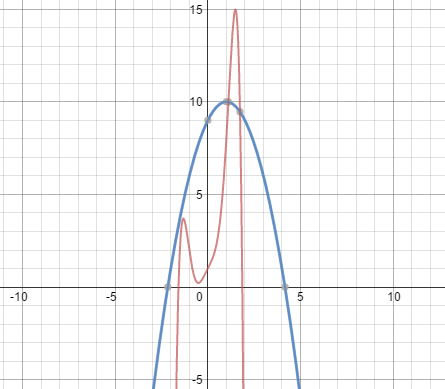3.5 In addition to Score 3.0 performance, the student demonstrates in-depth inferences and applications regarding the more complex content with partial success. 3.0 (proficient) The student can: identify key features in graphs and tables including intercepts; intervals where the function is increasing, decreasing, positive, or negative; relative maximums and minimums; symmetries; end behavior; domain and range; and periodicity for a given function. sketch the graph of a function given its key features. The student exhibits no major errors or omissions. Identify the following from the graph: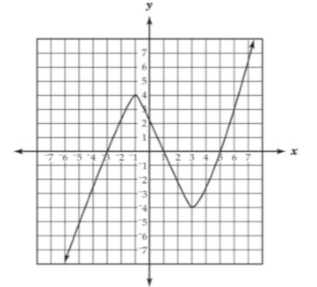x-intercepts: y-intercepts: Increasing Intervals: Decreasing Intervals: Positive Intervals: Negative Intervals: Maximums: Minimums: Domain: Range:   Sketch a function that would have an increasing interval from (3,+∞) and a relative minimum at (3,4). 2.5 The student demonstrates no major errors or omissions regarding the simpler details and processes (Score 2.0 content) and partial knowledge of the more complex ideas and processes (Score 3.0 content). 2.0 (progressing) There are no major errors or omissions regarding the simpler details and processes as the student can: Recognize and recall specific terminology such as: intervals intercept (x and y) relative minimum and maximum periodicity end behavior symmetry increasing decreasing use interval notation accurately. However, the student exhibits major errors or omissions regarding the more complex ideas and processes. Write the following in interval notation: x<3 x>5 x≥10 5

## Resources

 Web Vocab intercepts relative maximum relative minimum end behavior periodicity symmetry

#### MAT-HS.F-IF.05

 MAT-HS Targeted Standards(F) Concept: Function(IF) Domain: Interpreting FunctionsCluster: Interpret functions that arise in applications in terms of the context MAT-HS.F-IF.05 Relate the domain of a function to its graph and, where applicable, to the quantitative relationship it describes. For example, if the function h(n) gives the number of person-hours it takes to assemble n engines in a factory, then the positive integers would be an appropriate domain for the function.

## Student Learning Targets:

• I can
• I can

• I can
• I can

### Skills (Performance) Targets

• I can solve a linear system (substitution, linear combination, graphically).
• I can write a linear system.
• I can identify the number of solutions of a linear system.
• I can identify the most efficient method to solve a system.
• I can check the solution of the system.
• I can graph a linear equation.
• I can manipulate the form of a linear equation.
• I can identify a linear equation.
• I can interpret a graph to determine the appropriate numerical domain being described.

• I can
• I can

## Proficiency Scale

 Score Description Sample Activity 4.0 In addition to Score 3.0, the student demonstrates in-depth inferences and applications regarding more complex material that go beyond end of instruction expectations. - 3.5 In addition to Score 3.0 performance, the student demonstrates in-depth inferences and applications regarding the more complex content with partial success. 3.0 “The Standard.” The student demonstrates no major errors or omissions regarding any of the information and processes that were end of instruction expectations. - 2.5 The student demonstrates no major errors or omissions regarding the simpler details and processes (Score 2.0 content) and partial knowledge of the more complex ideas and processes (Score 3.0 content). 2.0 The student demonstrates no major errors or omissions regarding the simpler details and processes but exhibits major errors or omissions regarding the more complex ideas and processes (Score 3.0 content). - 1.5 The student demonstrates partial knowledge of the simpler details and processes (Score 2.0 content) but exhibits major errors or omissions regarding the more complex ideas and procedures (Score 3.0 content). 1.0 With help, the student demonstrates a partial understanding of some of the simpler details and processes (Score 2.0 content) and some of the more complex ideas and processes (Score 3.0 content). - 0.5 With help, the student demonstrates a partial understanding of some of the simpler details and processes (Score 2.0 content) but not the more complex ideas and processes (Score 3.0 content). 0.0 Even with help, the student demonstrates no understanding or skill. -

## Resources

 Web Vocab

Page:  1  2  3  4  (Next)
ALL